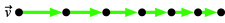# Problem: A partial motion diagram is shown in the figure above. Select the correct motion diagram with added acceleration vector.Select the correct physics problem for which this is the correct motion diagram.Select the correct pictorial representation for your problem.

###### Problem DetailsA partial motion diagram is shown in the figure above. Select the correct motion diagram with added acceleration vector.

Select the correct physics problem for which this is the correct motion diagram.

Select the correct pictorial representation for your problem.